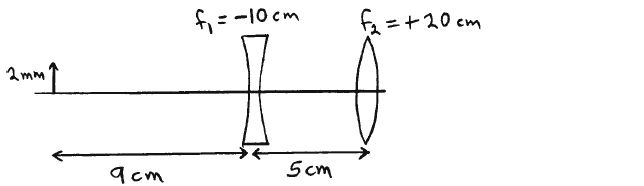# Problem: A diverging lens with  f1 = -10.0 cm is 5.0 cm to the left of a converging lens of   f2 = +20.0 cm. An object 2.0 mm tall is placed 9.0 cm to the left of the diverging lens. What is the distance of the final image from the converging lens?

###### FREE Expert Solution
90% (328 ratings)
###### Problem Details

A diverging lens with  f1 = -10.0 cm is 5.0 cm to the left of a converging lens of   f2 = +20.0 cm. An object 2.0 mm tall is placed 9.0 cm to the left of the diverging lens. What is the distance of the final image from the converging lens?